Free Printable Worksheets for Fourth grade

## General Statistics Worksheet – C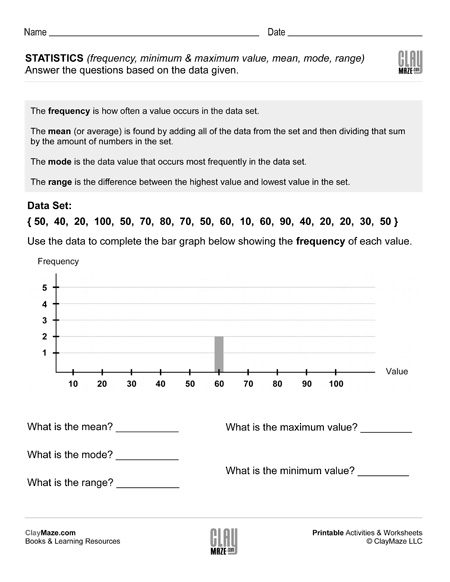The worksheet has statistics terms defined at the top for reference. Below that is a set of data values. Using these values, the student is asked to complete the graph. Following the graphs are...

## General Statistics Worksheet – BThere are statistics terms defined at the top of this worksheet followed by a data set and a graph to be filled out using the data set. Then the student asked questions about the data using the...

## General Statistics Worksheet – A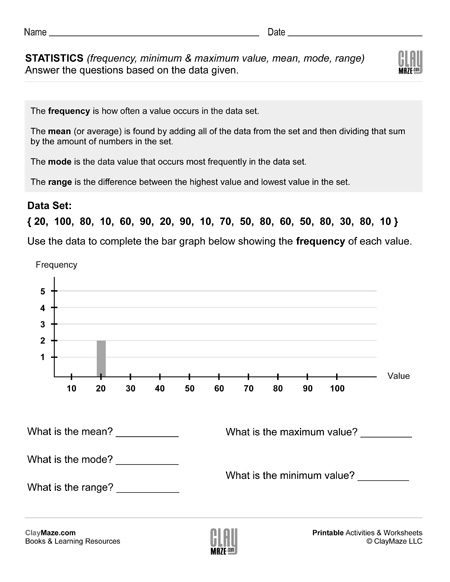This worksheet has a few statistics terms defined at the top. Following that is a data set and a graph to fill out using the data given. Below that are questions about the data which use the...

## Roman Numerals to Arabic Numerals: Higher NumbersRoman numerals free practice worksheet. Convert the Roman numerals to Arabic Numerals. – higher numbers Click here for our Roman numeral reference chart....

## Roman Numerals to Arabic Numerals: Lower Numbers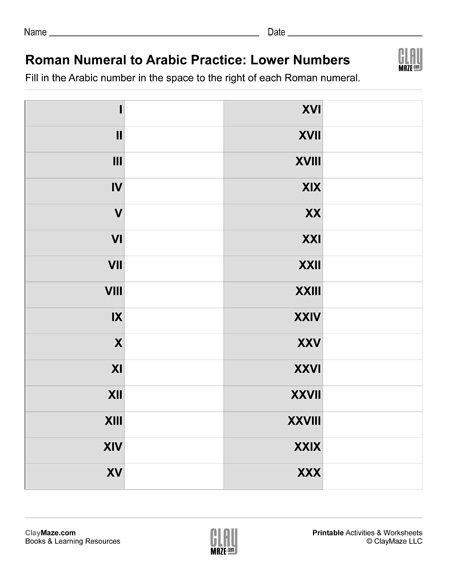Free Roman numerals worksheet. Write the Arabic numerals for each of the given Roman numerals. – lower numbers Click here for our Roman numeral reference chart....

## Arabic Numerals to Roman Numerals: Higher NumbersFree worksheet on Roman numerals. Write the Roman numerals for each of the given Arabic numerals. – higher numbers Click here for our Roman numeral reference chart....

## Arabic Numerals to Roman Numerals: Lower Numbers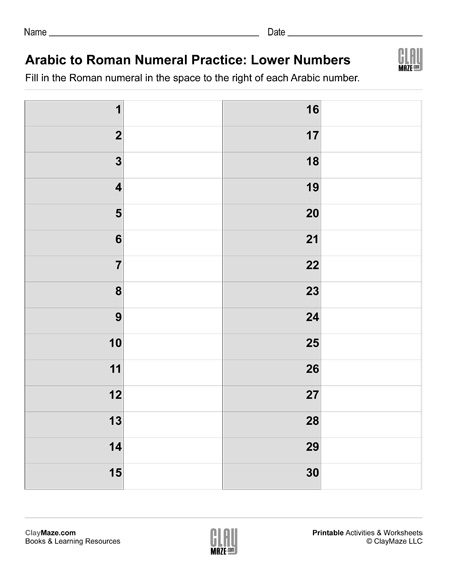Roman numerals free practice worksheet. Convert the Arabic numerals to Roman Numerals. – lower numbers Click here for our Roman numeral reference chart....

## Roman Numerals Reference ChartA reference chart of useful Roman numerals and their corresponding Arabic numerals. Download and print our Roman numeral worksheets: Arabic Numerals to Roman Numerals: Lower Numbers Arabic...

## Venn Diagrams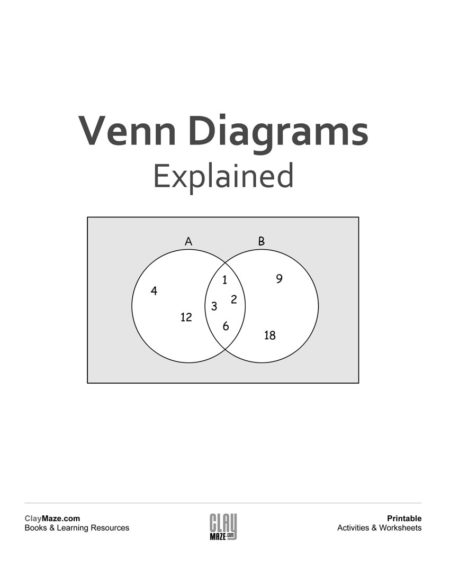A Venn diagram can be used to organize groups of things in a visual way to show how the groups are similar and how they are different. The similar objects of the groups are placed in the overlappin...

## Fill in the Venn Diagrams (Set 4)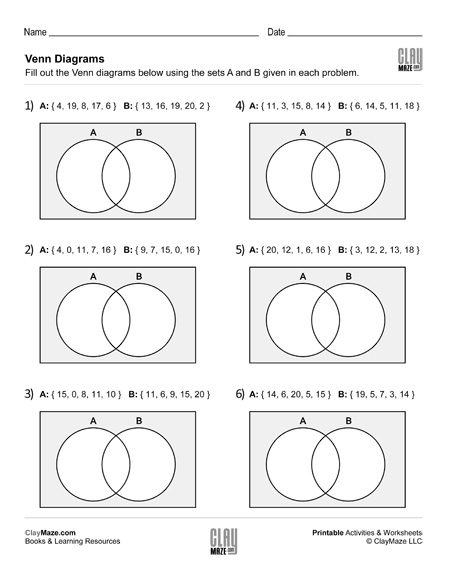Venn diagrams are a great exercise in learning how sets of numbers are related and how they differ. It also helps in data interpretation. For more explanation of what a Venn diagram is and to see...

## Fill in the Venn Diagrams (Set 3)Help your child develop an better understanding of number sets and how they are related as well as recording and reading data. For more explanation of what a Venn diagram is and to see an...

## Fill in the Venn Diagrams (Set 2)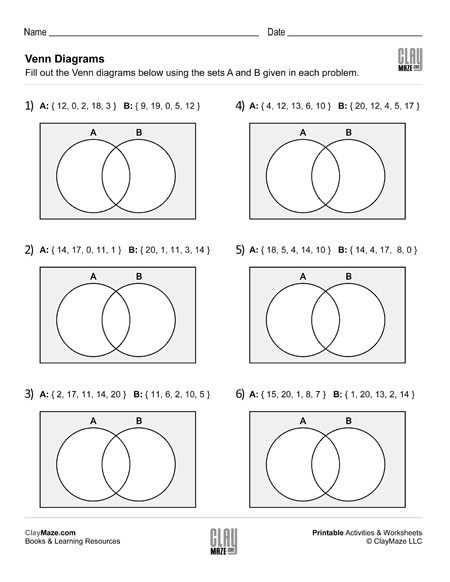Help your child develop an better understanding of logical relationships between  number sets. For more explanation of what a Venn diagram is and to see an example, click here....

## Fill in the Venn Diagrams (Set 1)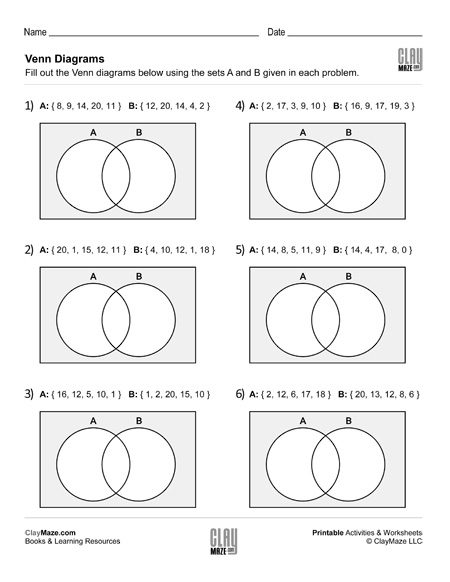Venn diagrams – Use the numbers sets to fill in the Venn diagrams. For more explanation of what a Venn diagram is and to see an example, click here.  ...

## Find the Missing Multipliers Worksheet (Set 4)Use this worksheet for practice in multiplication and division facts. – 20 problems...

## Find the Missing Multipliers Worksheet (Set 3)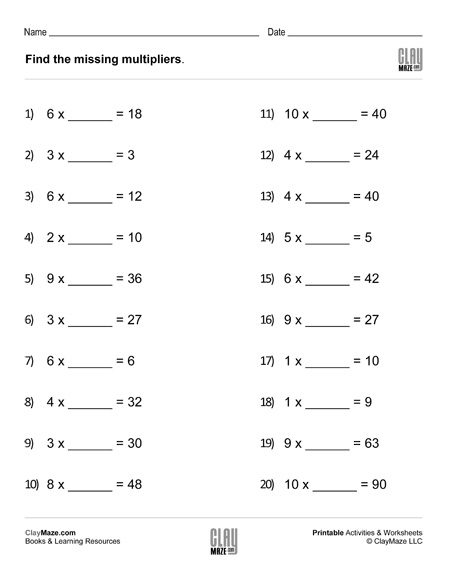Download this worksheet on finding missing multipliers to reinforce  knowledge of multiplication and division facts. – 20 problems...